﻿ 铸件凝固后期液膜抗拉能力分析
«上一篇文章快速检索 高级检索

 哈尔滨工程大学学报2019, Vol. 40Issue (6): 1182-1186  DOI: 10.11990/jheu.2018040010

### 引用本文LIU Yingwei, ZHANG Yang, DONG Xuefeng. Tensile ability of liquid film in the later stage of casting solidification[J]. Journal of Harbin Engineering University, 2019, 40(6), 1182-1186. DOI: 10.11990/jheu.201804001.### 文章历史

1. 哈尔滨工程大学 材料科学与化学工程学院, 黑龙江 哈尔滨 150001;
2. 伍伦贡大学, 机械、材料、机电一体化和生物医学工程系, 新南威尔士 2522

Tensile ability of liquid film in the later stage of casting solidification
LIU Yingwei 1, ZHANG Yang 1, DONG Xuefeng 2
1. School of Materials Science and Chemical Engineering, Harbin Engineering University, Harbin 150001, China;
2. School of Mechanical, Materials, Mechatronics and Biomedical Engineering, University of Wollongong, New South Wales 2522, Australia
Abstract: The later stage of metal solidification is a dangerous period for hot crack generation. Investigating the tensile strength of liquid films is important to improve casting quality given that the liquid film between crystal grains has a certain preventative effect on thermal cracking. The analytic formula is obtained through a calculus-based method, and the relationship between the radius of the curvature of the liquid film and grain spacing is first quantified based on mass conservation. Moreover, the indirect relationship between the tensile strength of the liquid film and grain spacing is obtained. Analytical results show that the radius of the curvature of the liquid film gradually decreases as the grain spacing is increased in the absence of liquid metal supplementation, and an extremum of the radius of curvature exists. However, the extreme value obtained in this study is different from that obtained in a previous study. The maximum of the tensile strength of the liquid film corresponds to the extremum of the radius of curvature. Crystal grains will not produce hot cracks if the shrinkage tension does not exceed this maximum value during solidification. This study provides an important reference for the establishment of the solidification process.
Keywords: solidification    surface tension    additional pressure    hot cracking    grain    grain boundary    curvature radius    tensile strength

1 液膜抗拉能力的计算 1.1 弯曲液面的附加压力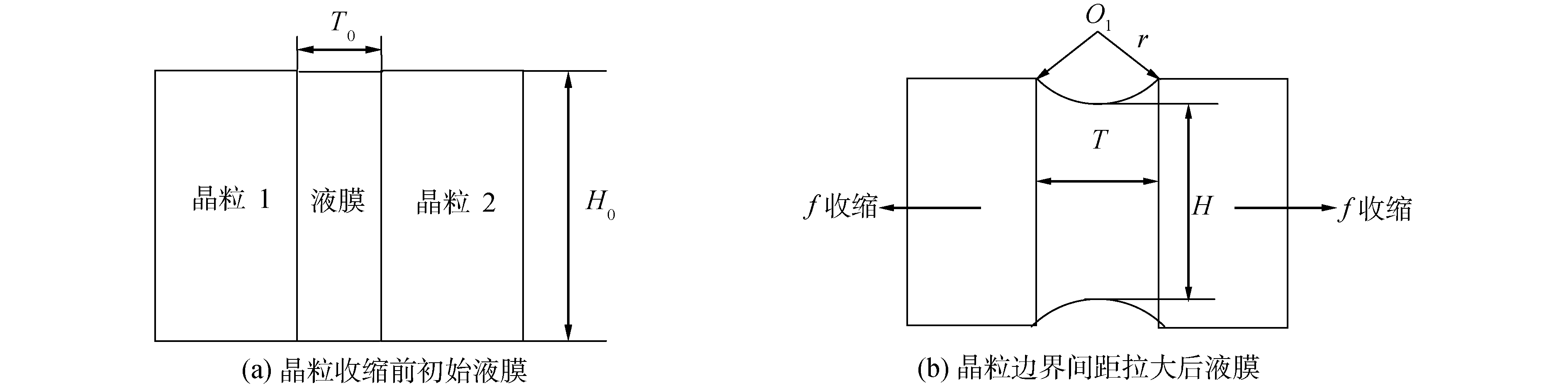Download: 图 1 液膜曲率半径与晶粒边界间距的关系 Fig. 1 Relationship between the radius of curvature of liquid film and grain spacing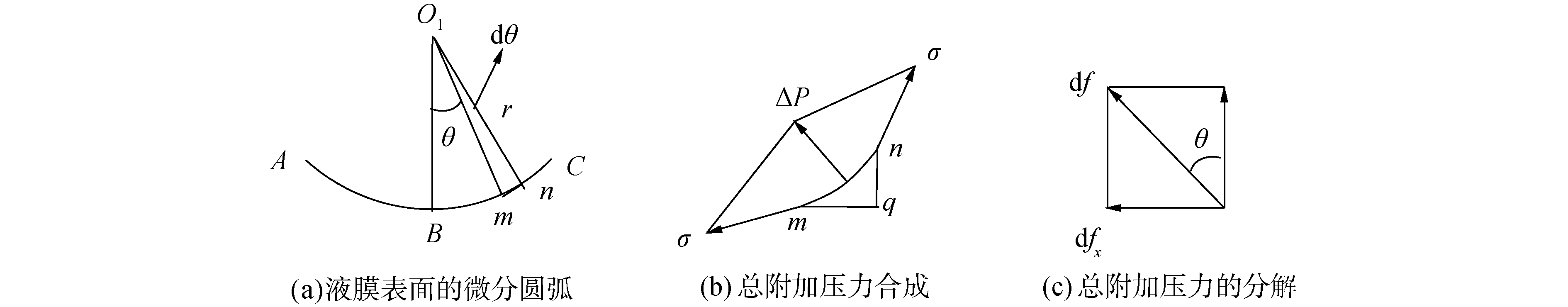Download: 图 2 液膜抗裂拉力的形成 Fig. 2 Formation of tensile strength of liquid film
1.2 液膜抗拉能力计算

 ${\rm{d}}f = \Delta P{\rm{d}}s$ (1)

 ${\rm{d}}{f_x} = {\rm{d}}f\sin \theta$ (2)

 ${\rm{d}}{f_x} = \frac{\sigma }{r}\sin \theta r{\rm{d}}\theta$ (3)

 $\begin{array}{l} {F_x} = \int\limits_B^c {\rm{d}} {f_x} = \int\limits_0^{{\theta _1}} {\frac{\sigma }{r}} \sin \theta r{\rm{d}}\theta = \\ - \sigma \cos \left. \theta \right|_{{\theta _1}}^0 = \sigma \left( { - 1 + \cos {\theta _1}} \right) \end{array}$ (4)

 ${\sigma _x} = {F_x}/\Delta y$ (5)

 $\Delta y = \int\limits_B^c {\rm{d}} y$ (6)

 $\Delta y = \left| {\int\limits_{{\theta _1}}^0 r \sin \theta {\rm{d}}\theta } \right| = | - r\cos \theta |_{{\theta _1}}^0| = r\left( {1 - \cos {\theta _1}} \right)$ (7)

 ${\sigma _x} = - (\sigma /r)$ (8)

2 液膜曲率半径与晶粒间隙的关系 2.1 质量守恒Download: 图 3 液膜的解析分析 Fig. 3 Analytical analysis of liquid film

 ${x^2} + {\left[ {y - \left( {r + \frac{H}{2}} \right)} \right]^2} = {r^2}$ (9)
 ${x^2} + {\left[ {y - \left( { - r - \frac{H}{2}} \right)} \right]^2} = {r^2}$ (10)

 ${y_1} = \left( {r + \frac{H}{2}} \right) - \sqrt {{r^2} - {x^2}}$ (11)
 ${y_2} = - \left( {r + \frac{H}{2}} \right) + \sqrt {{r^2} - {x^2}}$ (12)

 $S = \int_{ - \frac{T}{2}}^{\frac{T}{2}} {\left( {{y_1} - {y_2}} \right)} {\rm{d}}x$ (13)

 $S = \int_\limits{ - \frac{T}{2}}^{\frac{T}{2}} {\left[ {2\left( {r + \frac{H}{2}} \right) - 2\sqrt {{r^2} - {x^2}} } \right]} {\rm{d}}x$ (14)

 $S = 2\left( {r + \frac{H}{2}} \right)T - 2\left[ {\frac{T}{2}\sqrt {{r^2} - \frac{{{T^2}}}{4}} + {r^2}\arcsin \left( {\frac{T}{{2r}}} \right)} \right]$ (15)

 $\overline {{O_1}{K^2}} + \overline {K{C^2}} = \overline {{O_1}{C^2}}$

 ${(r - \Delta H)^2} + {\left( {\frac{T}{2}} \right)^2} = {r^2}$ (16)

 $\frac{H}{2} = \frac{{{H_0}}}{2} - r + \sqrt {{r^2} - {{\left( {\frac{T}{2}} \right)}^2}}$

 $S = T{H_0} + T\sqrt {{r^2} - \frac{{{T^2}}}{4}} - 2{r^2}\arcsin \left( {\frac{T}{{2r}}} \right)\$ (17)

 $T{H_0} + T\sqrt {{r^2} - \frac{{{T^2}}}{4}} - 2{r^2}\arcsin \left( {\frac{T}{{2r}}} \right) = {T_0}{H_0}$ (18)
2.2 曲率半径与晶粒边界间距的关系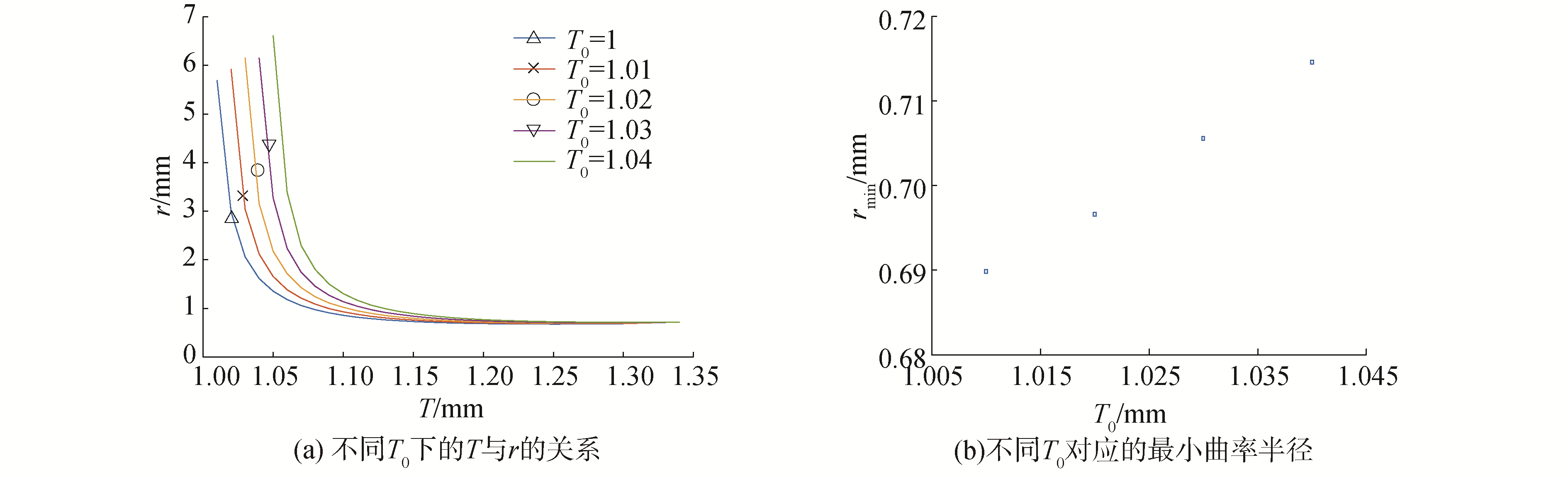Download: 图 4 晶粒边界间距与曲率半径的关系 Fig. 4 The relationship between grain spacing and radius of curvature

2.3 曲率半径与晶粒尺寸的关系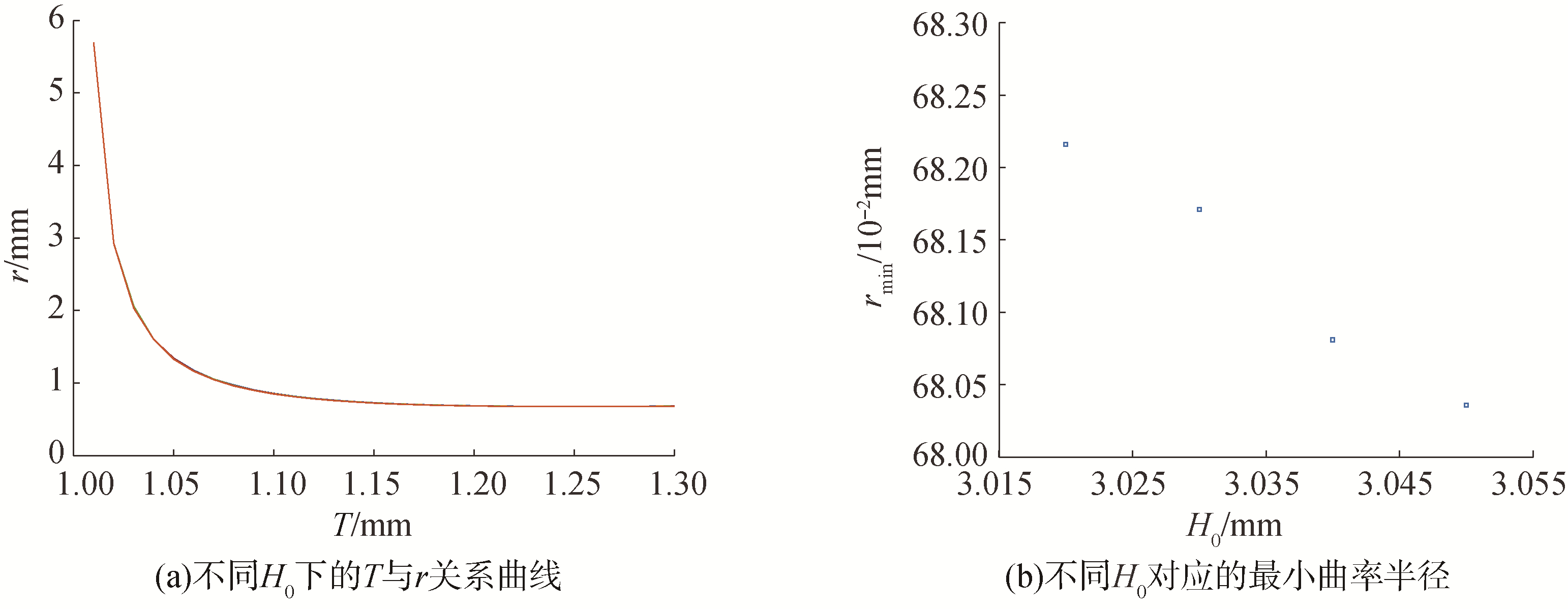Download: 图 5 晶粒尺寸与曲率半径的关系 Fig. 5 The relationship between grain size and radius of curvature
2.4 rmin的确定

 $\begin{array}{l} {H_0} + \sqrt {{r^2} - \frac{{{T^2}}}{4}} + T\frac{{2r\frac{{\partial r}}{{\partial T}} - \frac{T}{2}}}{{2\sqrt {{r^2} - \frac{{{T^2}}}{4}} }} - \left( {\frac{{\partial r}}{{\partial T}}} \right) \cdot \\ 4{\mathop{\rm rarcsin}\nolimits} \left( {\frac{T}{{2r}}} \right) - \frac{{\frac{1}{{2r}} - \frac{T}{{2\sqrt r }}\frac{{\partial r}}{{\partial T}}}}{{\sqrt {1 - {{\left( {\frac{T}{{2r}}} \right)}^2}} }}2{r^2} = 0 \end{array}$ (19)

 ${H_0}\sqrt {{r^2} - \frac{{{T^2}}}{4}} - \frac{{{T^2}}}{2} = 0$

 ${r_{\min }} = \sqrt {\frac{{{T^2}}}{4} + \frac{{{T^4}}}{{4H_0^2}}}$ (20)
2.5 分析与讨论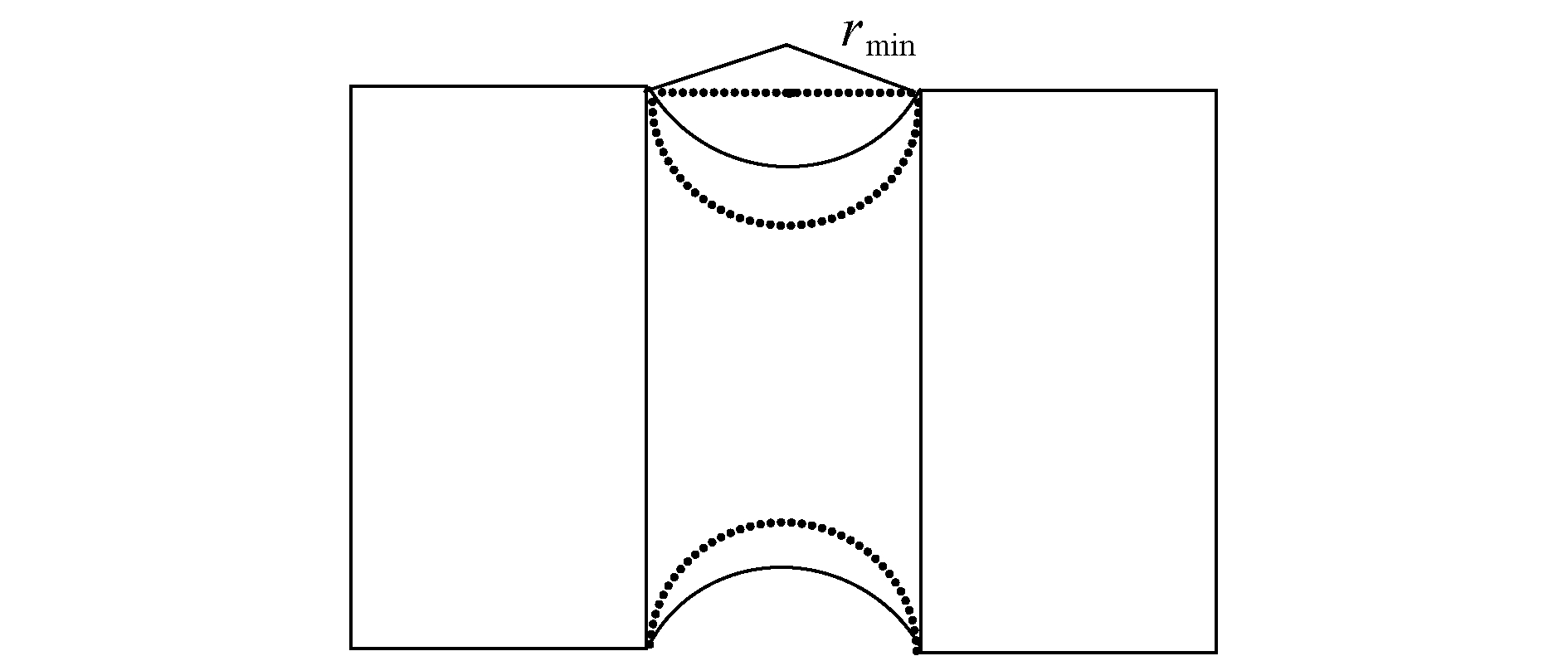Download: 图 6 传统最小曲率半径与本文最小曲率半径比较示意 Fig. 6 A comparison between the traditional and currently calculated minimum radius of curvature

3 结论

1) 本文得到的液膜最小曲率半径与传统理论不同，传统的最小曲率半径未涉及晶粒尺寸，而本文则考虑了这一点。

2) 分析表明，晶粒尺寸对最小曲率半径有影响，进而影响液膜抗拉能力，这一结果更接近实际情况，可为实际的凝固工艺的制定提供有意义的参考。

  陈德平, 许健, 杨刚, 等. 不同浇注方式下铸造风机主轴的热裂模拟及工艺优化[J]. 金属铸锻焊技术, 2011, 40(7): 54-56, 62. CHEN Deping, XU Jian, YANG Gang. Simulation of hot tearing of fan main shaft casting under different pouring methods and its process optimization[J]. Casting·forging·welding, 2011, 40(7): 54-56, 62. (0)  谢正茂, 阎顺祥, 赵小军, 等. NT缸体铸造裂纹产生原因分析[J]. 热加工工艺, 2017, 46(5): 243-247. XIE Zhengmao, YAN Shunxiang, ZHAO Xiaojun, et al. Analysis on causes of casting cracks in NT cylinder block[J]. Hot working technology, 2017, 46(5): 243-247. (0)  虞强, 彭家锋, 刘国江. 铸造起重机吊具横梁裂纹原因及改造措施[J]. 机电工程技术, 2017, 46(8): 203-205. YU Qiang, PENG Jiafeng, LIU Guojiang. The reasons and measures of casting crane beam crack[J]. Mechanical & electrical engineering technology, 2017, 46(8): 203-205. DOI:10.3969/j.issn.1009-9492.2017.08.060 (0)  宋平, 常伟, 苑英伟, 等. 5083铝合金大规格扁锭铸造热裂纹缺陷的研究[J]. 有色金属加工, 2016, 45(5): 22-25. SONG Ping, CHANG Wei, YUAN Yingwei, et al. Study of hot cracking in casting of large-size 5083 aluminum alloy ingot[J]. Nonferrous metals processing, 2016, 45(5): 22-25. DOI:10.3969/j.issn.1671-6795.2016.05.005 (0)  石照夏, 董建新, 张麦仓. 汽车增压器涡轮用铸造高温合金热裂研究进展[J]. 材料工程, 2012, 40(6): 91-96. SHI Zhaoxia, DONG Jianxin, ZHANG Maicang. Development of hot tearing on cast superalloys used for auto turbocharger turbine wheel[J]. Journal of materials engineering, 2012, 40(6): 91-96. DOI:10.3969/j.issn.1001-4381.2012.06.020 (0)  XU Rongfu, ZHENG Hongliang, LUO Jie, et al. Role of tensile forces in hot tearing formation of cast Al-Si alloy[J]. Transactions of nonferrous metals society of China, 2014, 24(7): 2203-2207. DOI:10.1016/S1003-6326(14)63333-1 (0)  祖方遒, 袁晓光, 梁维中. 铸件成形原理[M]. 北京: 机械工业出版社, 2013: 28-29. (0)  刘文君, 蒋斌, 杨青山. 双辊铸造热裂形成及工艺控制[J]. 材料导报A:综述篇, 2011, 25(9): 126-130. LIU Wenjun, JIANG Bin, YANG Qingshan. Hot tearing formation and control in twin-roll casting[J]. Materials review, 2011, 25(9): 126-130. (0)